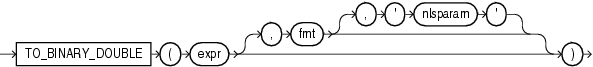# TO_BINARY_DOUBLE

SyntaxDescription of the illustration to_binary_double.gif

Purpose

`TO_BINARY_DOUBLE` returns a double-precision floating-point number.

• `expr` can be a character string or a numeric value of type `NUMBER`, `BINARY_FLOAT`, or `BINARY_DOUBLE`. If `expr` is `BINARY_DOUBLE`, then the function returns `expr`.

• The optional '`fmt`' and '`nlsparam`' arguments are valid only if `expr` is a character string. They serve the same purpose as for the `TO_CHAR` (number) function.

• The case-insensitive string '`INF`' is converted to positive infinity.

• The case-insensitive string '-`INF`' is converted to negative identity.

• The case-insensitive string '`NaN`' is converted to `NaN` (not a number).

You cannot use a floating-point number format element (`F`, `f`, `D`, or `d`) in a character string `expr`.

Conversions from character strings or `NUMBER` to `BINARY_DOUBLE` can be inexact, because the `NUMBER` and character types use decimal precision to represent the numeric value, and `BINARY_DOUBLE` uses binary precision.

Conversions from `BINARY_FLOAT` to `BINARY_DOUBLE` are exact.

Examples

The examples that follow are based on a table with three columns, each with a different numeric datatype:

```CREATE TABLE float_point_demo
(dec_num NUMBER(10,2), bin_double BINARY_DOUBLE, bin_float BINARY_FLOAT);

INSERT INTO float_point_demo
VALUES (1234.56,1234.56,1234.56);

SELECT * FROM float_point_demo;

DEC_NUM BIN_DOUBLE  BIN_FLOAT
---------- ---------- ----------
1234.56 1.235E+003 1.235E+003
```

The following example converts a value of datatype `NUMBER` to a value of datatype `BINARY_DOUBLE`:

```SELECT dec_num, TO_BINARY_DOUBLE(dec_num)
FROM float_point_demo;

DEC_NUM TO_BINARY_DOUBLE(DEC_NUM)
---------- -------------------------
1234.56                1.235E+003
```

The following example compares extracted dump information from the `dec_num` and `bin_double` columns:

```SELECT DUMP(dec_num) "Decimal",
DUMP(bin_double) "Double"
FROM float_point_demo;

Decimal                     Double
--------------------------- ---------------------------------------------
Typ=2 Len=4: 194,13,35,57   Typ=101 Len=8: 192,147,74,61,112,163,215,10
```